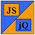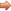# JavaScript & jQuery Tutorials

JS HomeJS BasicsBasic Maths Functions

## Basic Maths Functions

In this lesson we look at some of the basic maths and assignment operators. Basic mathematical operations are supported in JavaScript using symbols as follows:

• Addition uses the + symbol as in 10 + 10
• Subtraction uses the - symbol as in 10 - 10
• Division uses the / symbol as in 10 / 10
• Multiplication uses the * symbol as in 10 * 10
• Modulus uses the % symbol as in 17 % 5

You can also assign numbers to variables and apply maths to them.

``````
// Create some variables and add them together.
var firstNumber = 10;
var secondNumber = 10;

``````

Press the button below to action the above code:

### Operation Order.

There are certain rules to follow when performing several mathematical operations in the same statement.

• Use parentheses to group operations you want performed first.
• Orders (also known as indices and exponents) which are powers and square roots etc. are appplied next.
• Division and Multiplication take precedence over Addition and Subtraction.
• After these precedences are applied maths is worked out from left to right.

``````
/*
* No Parentheses so multiplication will be done first.
* Result is 32 not 50
*/
alert(2 + 3 * 10) + ' NO Parentheses so multiplication will be done first';

// Parentheses will be done first.
alert((2 + 3) * 10) + ' Maths in Parentheses will be done first';

``````

Press the button below to action the above code:

### Maths Shortcut Assignments For Variables

There are several shortcuts available when performing maths on a variable.

• += will add the value on the right of the equal sign to the variable of the left.
• -= will subtract the value on the right of the equal sign from the variable on the left.
• *= will multiply the value on the right of the equal sign by the variable on the left.
• /= Divides the variable by value on the right of the equal sign.
• ++ Placed after a variable will add 1 to the variable.
• -- Placed after a variable will subtract 1 from the variable.

``````
aTotal = 0;
aTotal = aTotal + 10;
aTotal += 10; // Same as above

aTotal = aTotal * 10;
aTotal *= 10; // Same as above

aTotal = 0;
aTotal++; // aTotal equals 1

``````

## Lesson 5 Complete

In this lesson we looked at some of the basic mathematical functions, precedence and assignment shortcuts.

All the arithmetic operators are discussed in JavaScript Reference - Arithmetic Operators and the assignment operators are discussed in JavaScript Reference - Assignment Operators. So any operators not covered here will be discussed in those references.

## What's Next?

In the next lesson we look at variable usage in JavaScript.